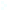Election CitationResearch Interests Name Bhargava, Manjul Location Princeton University Primary Field Mathematics Bhargava has quickly established himself a world leader in number theory. His unexpected discoveries of algebraic structures in the diophantine theory of homogeneous spaces led him to the solution of a number of fundamental problems concerning such basic objects in number theory as algebraic number fields and elliptic curves. Using varied techniques from algebra, analysis, and geometry, Bhargava addresses basic questions in number theory. Examples include: when does a positive-definite quadratic form with integer coefficients take every possible positive integer value? What is the probability that an elliptic curve equation y^2 = x^3 + A x + B has a rational solution (x,y)? What is the probability that a random integer polynomial of large degree takes a square value? Bhargava uses such questions as a starting point to study the structure of the integers, higher degree polynomials and forms over the integers, and related arithmetic and geometric objects.